GBP

## Paper Pencil Geometry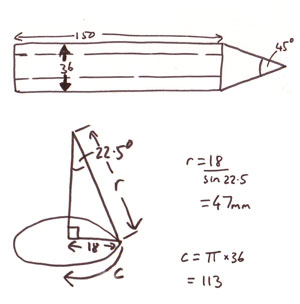Here's a quick mathematical diversion. How would you make a pencil from paper? How do you join the hexagonal section of the shaft to the cone of the point? As is often the case, geometry has the answer.

My starting point is to create a pencil with the dimensions shown top left. (all dimensions are in millimeters)

First step, the cone. The radius of the base of the cone is 18mm, half of the 36mm diameter of the shaft.

I've picked an angle of 45° for the pencil point so the angle of the inside triangle is half of that, 22.5°

The length 'r' is 47mm, worked out using a sine.

The length all the way round the base of the cone 'C' is 113mm. Remember those numbers, we'll be coming back to them.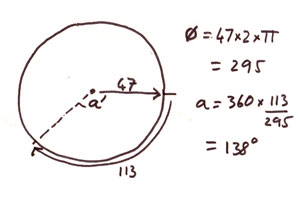The cone is made from a pie slice of card mathematically know as a sector. The angle of the slice determines the angle of the finished cone. The wider the angle, the flatter and less pointed the cone.

A full circle with diameter 47mm has a circumference of 295mm. Using this and the length C from before it turns out the angle of pie that we need is 138°

Nearly there! Keep up at the back!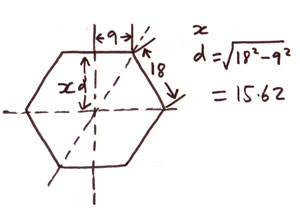We'll need some scallop shapes to link the hexagon tube with the cone. One more bit of calculation is needed to find the size of the scallop.

Time to wheel out Pythagoras giving a value for x of 15.61mm.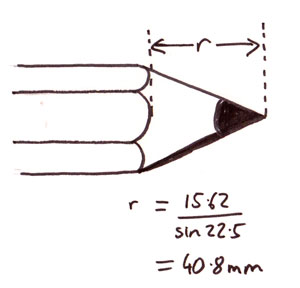Using the same technique as before the new value for r, the distance from the point of the cone to the end of the scallops is 40.8mm

Having worked out all these dimensions I was delighted that the whole thing just slotted together nicely. I love the power of mathematics and geometry to work out how shapes fit together, its like having magic powers! Wooo!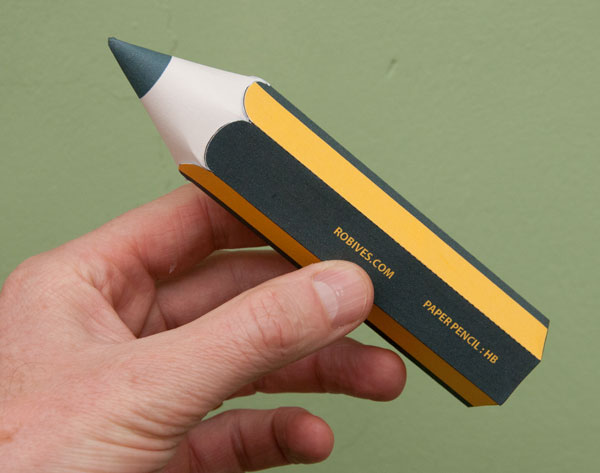I'll be putting up the parts as a download shortly. Nessie next.

Ooo look! Post 900!